# ISEE Middle Level Math : How to find median

## Example Questions

### Example Question #11 : How To Find Median

Subtract the mode from the median in this set of numbers:

9080, 9008, 9800, 9099, 9009, 9090, 9008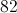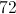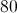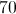Explanation:

First, order the numbers from least to greatest: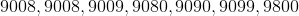Then, find the mode (the most recurring number): 9008

Then, find the median (the middle number):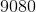Finally, subtract the mode from the median: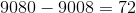### Example Question #12 : How To Find Median

Find the median in this set of numbers: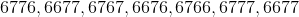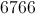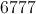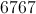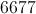Explanation:

First, order the numbers from least to greatest: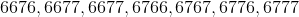Then, identify the middle number: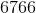### Example Question #13 : How To Find Median

Find the median in this set of numbers:

5855, 5588, 5585, 5858, 5885, 5558, 5885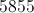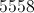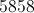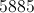Explanation:

First, order the numbers from least to greatest: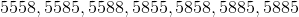Then, find the middle number: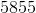.

### Example Question #14 : How To Find Median

Find the median in this set of numbers:

787, 778, 878, 877, 778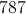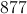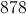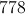Explanation:

First, order the numbers from smallest to largest: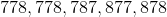Then, identify the middle number: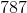Answer: The median is.

### Example Question #15 : How To Find Median

Find the median in this set of numbers: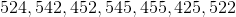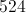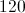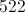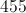Explanation:

First, order the numbers from least to greatest: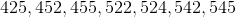Then, identify the  middle number: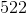### Example Question #16 : How To Find Median

Find the median of this set of numbers: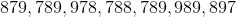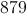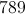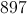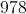Explanation:

First, order the numbers from least to greatest: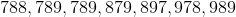Then, identify the middle number: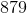### Example Question #17 : How To Find Median

Find the median of this set of numbers: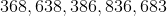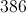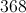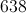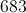Explanation:

The median of a data set is the middle number.

First, order the numbers from least to greatest: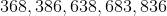Then, identify the middle number: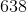### Example Question #18 : How To Find Median

Find the median of this set of numbers: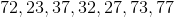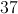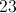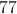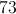Explanation:

The median of a data set is the middle number.

First, order the numbers from least to greatest: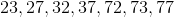Then, identify the middle number: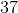### Example Question #19 : How To Find Median

Find the median of this set of numbers: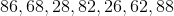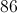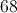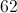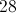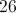Explanation:

First, order the numbers from least to greatest: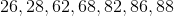Then, identify the middle number: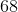### Example Question #20 : How To Find Median

Find the median of this set of numbers: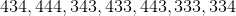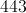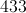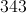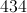Explanation:

First, order the numbers from least to greatest: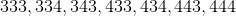Then, identify the middle number: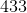### All ISEE Middle Level Math Resources